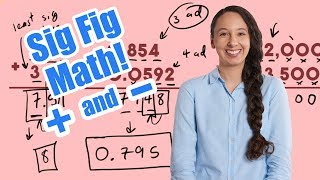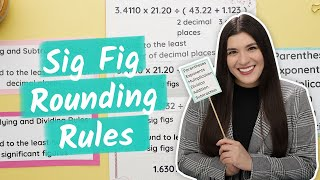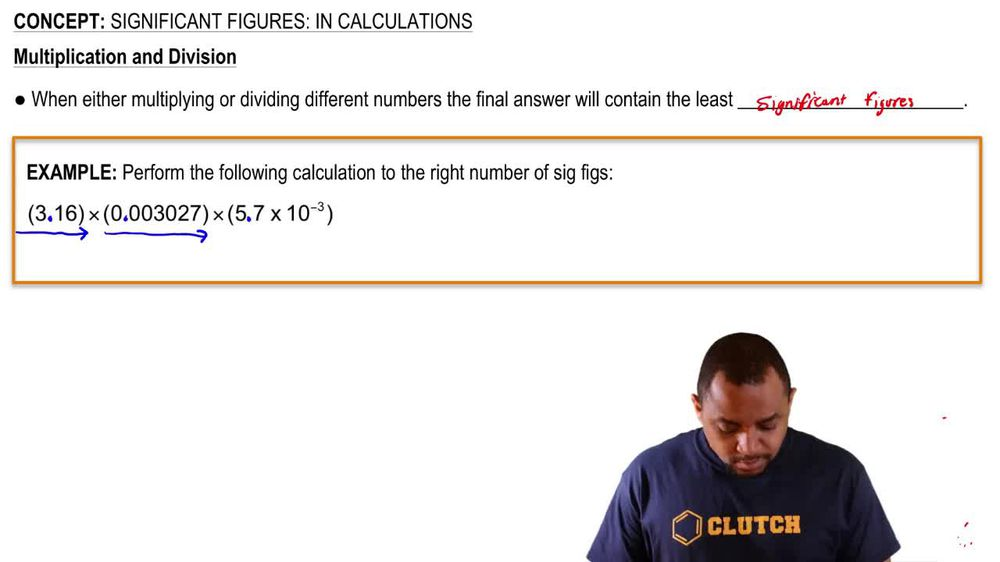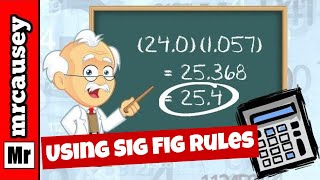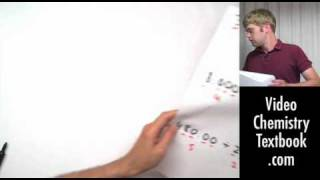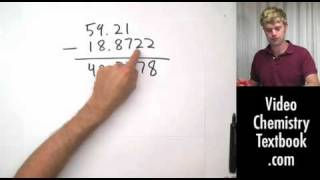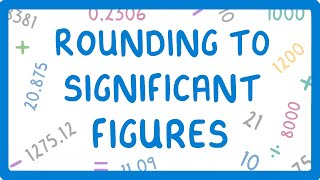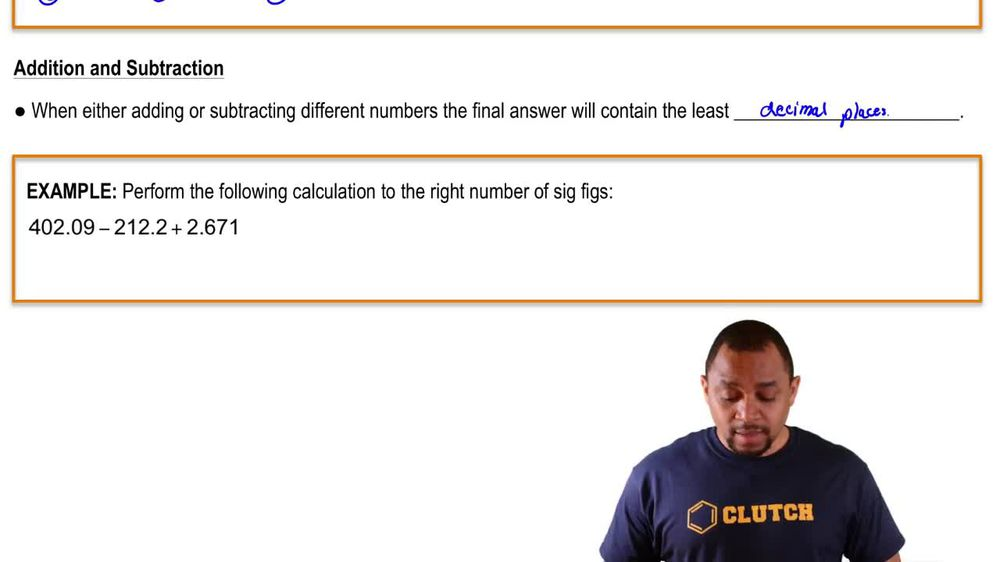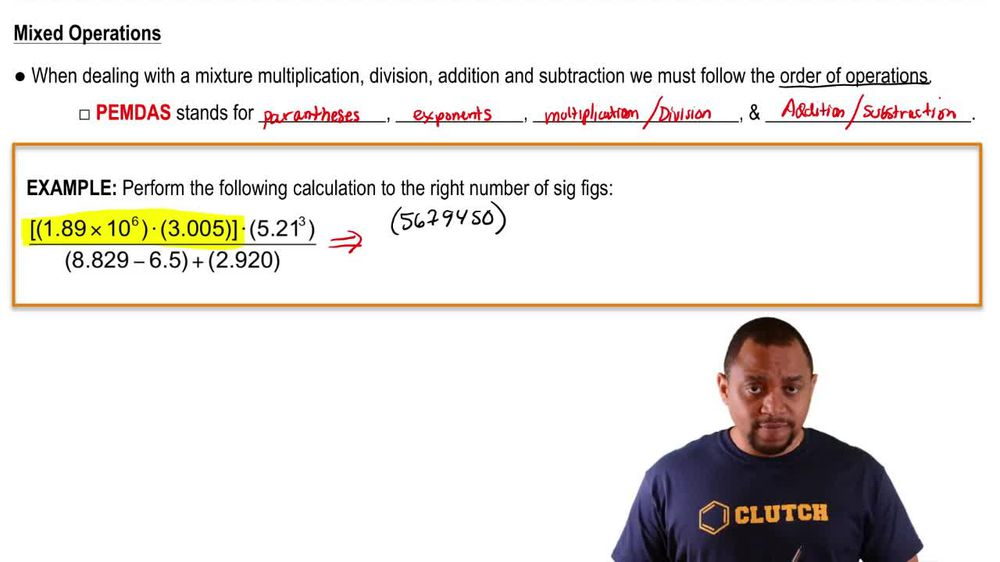Start typing, then use the up and down arrows to select an option from the list.
1. 1. Intro to General Chemistry2. Significant Figures: In Calculations
Problem

# What answer should be reported, with the correct number of significant figures, for the following calculation?[(42.00 − 40.914) ⋅ (25.739 − 25.729)] / [(11.50⋅1.001) + (0.00710 ⋅ 700.)]

Next question